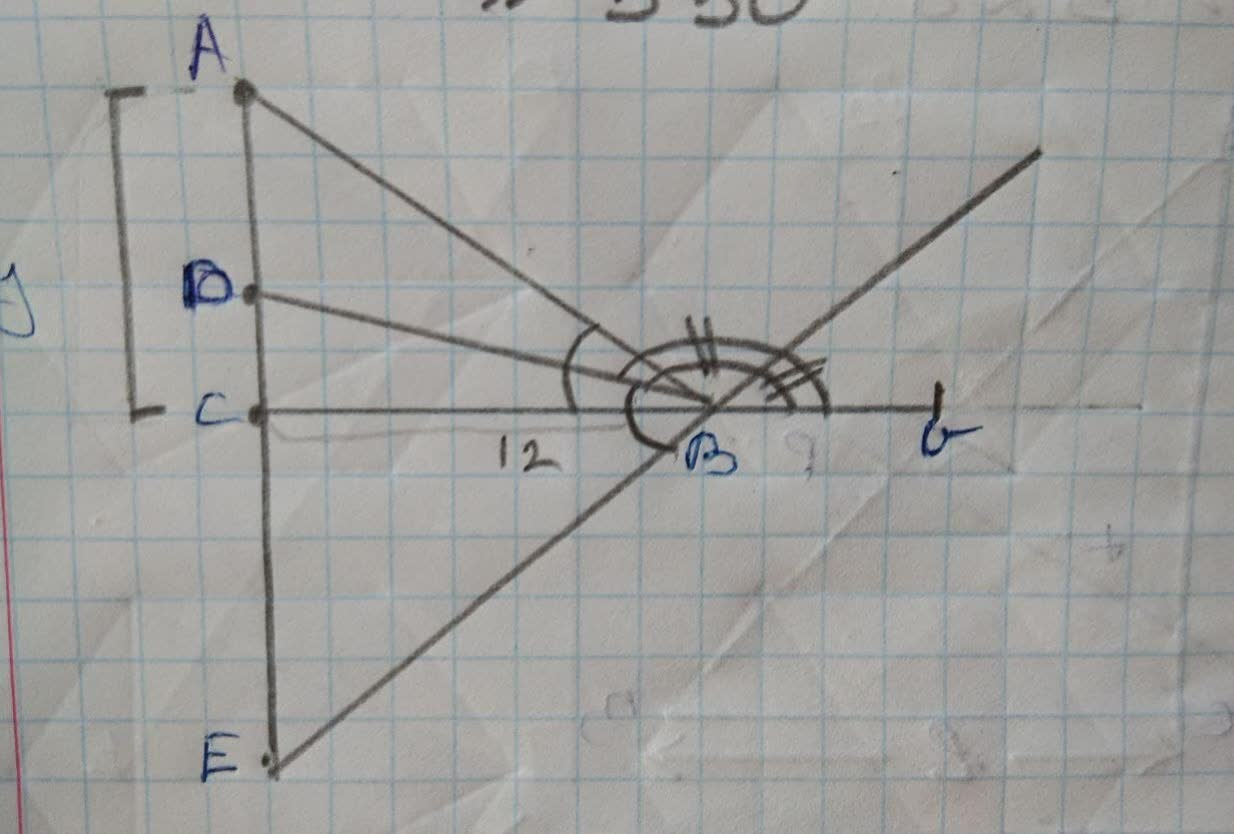## triangle ABC is a right triangle

Trigonometrytriangle ABC is a right triangle2 years ago
triangle ABC is a right triangle with its right angle at C. The bisector of angle B intersects AC at D. The bisector of the exterior angle at B intersects AC at E. If BD= 15 and BE=20, what are the lengths of triangle ABC?
Step 1 Using basic properties of triangles we can solve this question.Step2 angle abc is bisected by bd angle abg is bisected by be angle dbe=90(half of a angle in a straight line) bd=15cm be=20cm By pythagoras theorem, de=25cm Let de=xcm be=sqrt(be^(2)-ce^(2)) =sqrt(bd^(2)-dc^(2)) =12cm Step 3 ∠ dbc=(dc)[bc) =(9)/(12) dc=x ec=25-x be^(2)-ce^(2)=bd^(2)-x^(2) x=dc =9cm ∠ abc=2∠ 0bc =(24)/(7) (24)/(7)=(y)/(12) y=(24* 12)/(7) ac=(288)/(7) By pythagoras theorem ac^(2)+bc^(2)=ab^(2) ab=(300)/(7)